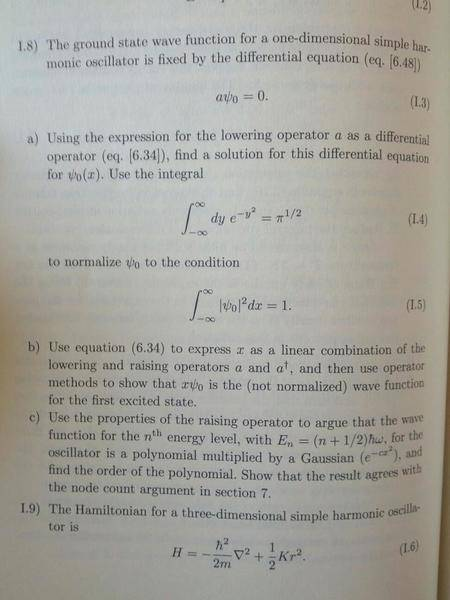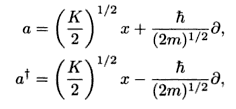# Ladder operators and a 1-D QHO

gronke

## Homework Statement## Homework Equations## The Attempt at a Solution

I solved part a) correctly, I believe, giving me

ψ = e$^{-(√(km)/\hbar)x^{2}}$

and a normalization constant A = ((π$\hbar$)/(km))$^{-1/4}$

I'm having difficulty with part b. I'm not exactly sure how I create a linear combination. I think I'm supposed to write the linear combination like so:

a + a$^{+}$ = 2(k/2)$^{1/2}$x

(a + a$^{+}$)(2k$^{-1/2}$) = x

However, I am not sure how I use the operator methods to show that xψ0 is the first excited state.

If anyone could nudge me in the right direction, I'd be eternally grateful! Thank you.